#Function Repository Resource:

# FindHypergraphIsomorphism

Find all isomorphisms between two orderless (undirected) hypergraphs

Contributed by: Jonathan Gorard
 ResourceFunction["FindHypergraphIsomorphism"][h1,h2] finds all possible isomorphisms that map the hypergraph h1 to h2 by renaming vertices.

## Details and Options

Hypergraphs are specified as lists of hyperedges.
ResourceFunction["FindHypergraphIsomorphism"] gives an association of the form Association[v1{w1,},v2{w2,}], where vi are vertices in h1 and wj are corresponding vertices in h2.
ResourceFunction["FindHypergraphIsomorphism"] gives an empty association if no isomorphism can be found.
Two hypergraphs are isomorphic if there exists a renaming of vertices that makes them identical.
The algorithm used in ResourceFunction["FindHypergraphIsomorphism"] is a direct generalization of Gorard’s uniqueness tree algorithm for standard graph isomorphism: https://arxiv.org/abs/1606.06399.

## Examples

### Basic Examples (3)

Find all isomorphisms between two hypergraphs:

 In:=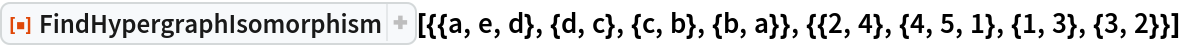Out=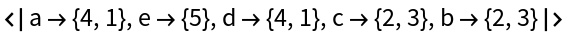Determine that two hypergraphs are not isomorphic:

 In:=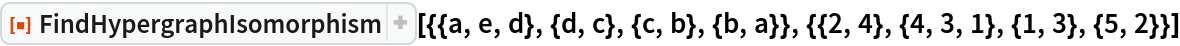Out=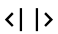Lists of hyperedges that are isomorphic as orderless hypergraphs may not be isomorphic as ordered hypergraphs:

 In:=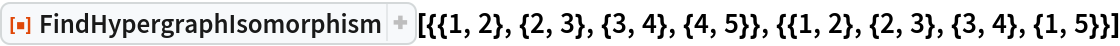Out=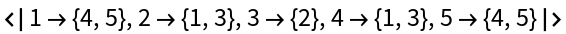### Scope (2)

FindHypergraphIsomorphism also works for standard undirected graphs:

 In:=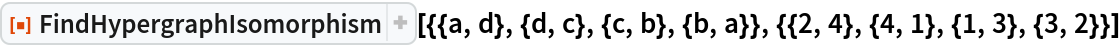Out=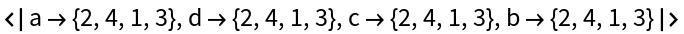In this case, it is functionally equivalent to :

 In:=Out=